Disclaimer: This is an example of a student written essay.

Any scientific information contained within this essay should not be treated as fact, this content is to be used for educational purposes only and may contain factual inaccuracies or be out of date.

# Slowing Down the Speed of Light

 ✅ Paper Type: Free Essay ✅ Subject: Physics ✅ Wordcount: 4304 words ✅ Published: 30th Jan 2018

STOPPING LIGHT IN ITS TRACKS

• Noorul Husna Binti Safian Sauri

Abstract

Introduction

The fact that speed of light which is very fast can be slowed down and stopped when there is change in refractive index. Stopped light achieved when the optical pulses are trapped in some material such as in the experiment which have been done by G. Heinze et al.. In that experiment, the optical pulses was stopped in cooled Pr3+:Y2SiO5 crystal up to 20µs. Then slow light can be obtained by measured the decrease in group velocity. A.V Turukhin et al. done the experiment to observed the ultraslow light. As a result from their experiment, a 45ms-1 speed of light were observed.

The group velocity, is defined as vg(w) = c / n(w) and from the equation ng(w) = n(w) + w dn (w)/dw , it showed the slow light can be obtained from the variation of refractive index and the dispersion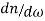of the material. Furthermore, when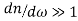, the group velocity can be highly reduced in material. So in order to achieve the slow light, material with higher dispersion is needed. However, in this situation a strong absorptions have become a big obstacle. It is because of the fact that higher dispersion gives a higher absorption and lead to a bad data transmission.

Technique of Electromagnetically Induced Transparency (EIT) introduced by researchers give the best solution for the strong absorptions although the EIT does not give 100% transmission. At the same time, EIT give additional advantages in order to slowed down and stopped the fast speed optical pulses.

A solid-state medium is more preferred in many applications of slow light because it has special properties. In solid- state medium, atomic diffusion is not being present, so it allow longer times for optical pulses storage. The slow light techniques play important role in numerous applications for example in optical- communication system.

Theoretical background

The condition of vg << c always refers to the phenomena of slow light. By using this condition, S.E. Harris et al. have observed the group velocities less than 17ms-1 in their experiment.In order to obtain the slow light, I need to understand the basic concept of phase velocity and group velocity. The phase velocity in a dispersion medium is defined as the velocity of points which have a constant phase. These velocity refer to the propagation of the constant phase of any one frequency of the wave. This wave can be shown by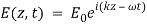(1)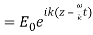(2)

Where E is electric field and ω is angular frequency propagating through a medium. Then the phase of this wave is given by :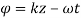(3)

The points of constant phase will move in a velocity of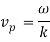(4)

where k is a wavenumber. Then used the relation of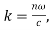the phase velocity become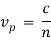(5)

. n from the equation is refer to a refrective index and c is the velocity of light in a vacuum. When the field is not considered as monochromatic, that velocity is defined as a group velocity and this velocity shows the propagation rate of changes in amplitude. Mathematically, group velocity is given by :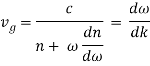(6)

Group velocity also can be defined as velocity at which energy or information is transmitted along a wave. Noticed that group index is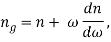(7)

then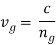(8)

From equation (6), the group index depends on dispersionand slow light can be obtained by making the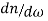large.

Professor Jakob Khanin and Olga Kocharovskaya were the first physicists that introduced the theory of EIT. EIT is a phenomenon of quantum optics which permits an opaque medium to be transparent to the optical light wave while still keeping the strong dispersion needed to generate the slow light. Based on Figure 2, when a first light beam is applied to the opaque medium, the light beam is completely absorbed. Then, after a second light is applied to the opaque medium suddenly the medium become transparent.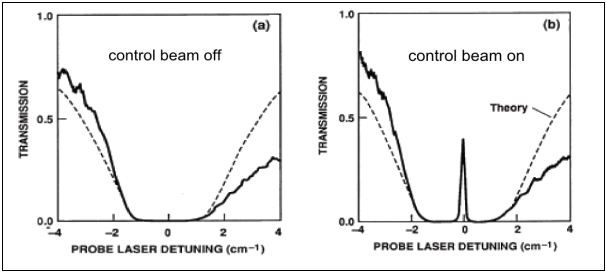Figure 1: observation when the control beam is off and on

The atoms experience a spontaneous process. When light beam comes in which it resonant with the frequency of the transitions, the atoms will excite to the higher energy level. This situation describes the normal absorption of light. Then, without any external radiation, the atom will decay spontaneously and end up in the lower state. These process depend on the number density of photon,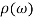. At first, I study the two level atomic system. From Einstein B coefficients, the transition probability up from ground state |1> to excited state |2> is B12and from the Einstein A coefficient, the spontaneous transition down from excited state |2> to ground state of |1> is A21. When the light is shining in the system, the number N1 of atom at the state |1> must be equal with the population N2 of atoms in the state |2>. Then, the absorption in the system is said to be saturated which mean there is no absorption of light beam can be happen. This situation will not be able to make the phenomena of EIT from being happen. To make the two level system unbalance, another state is introduce by shining a second light. This is why three level atomic system is used in the EIT.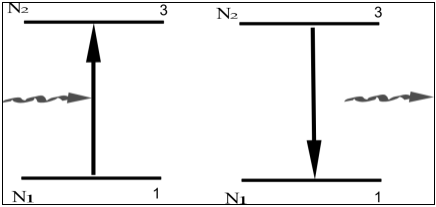Figure 2 : representation of absorption and spontaneous emission

Figure 3 illustrates the three level atomic system interact by a control beam which is a strong optical field and a probe beam which is a weak optical field. The three level system is also called a á´§-system based on its shape. The atoms are consider to have a pair of lower energy states of |1> and |2>. The probe beam couples states |1> and |3> with frequency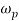while the control beam couples states |2> and |3> with frequency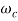. The two pathway interfere and cancel each other and then create a dark states polariton. Dark states means that there is no atoms will be promote to the excited state and then vanish the absorption of light.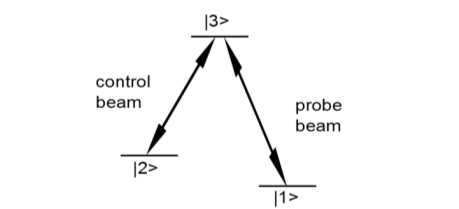Figure 3 : representation of three level atomic system

The expression of linear susceptibility spectrum explained the how the atomic ensemble respond to the strong resonant control beam and weak probe beam. The expression is :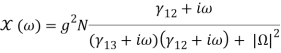(9)

Where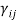be similar to the relaxation rate of the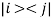coherence, N is the total number of atoms in the sample,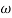is the difference between the probe beam frequency and the frequency of the atomic transition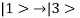(with= 0 show the frequency of the atom-field resonance),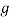is the atom-field coupling constant, and lastly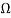is the Rabi frequency of the control beam[]. Rabi frequency is defined as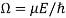, where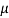and E are correspond to the transition moment and electric field of the control beam[].From the susceptibility, the imaginary part,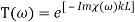(10)

where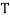is the transmission coefficient describes absorptive properties of the medium and L is the length of the medium. Then the real part of the susceptibility,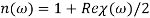(11)

showed the refractive index. Based on figure 4, the various ‘wiggles’ refractive index which

is green line showed the behaviour of nonlinear when detuning the frequency. The strength of the dispersion which relate to the slow light is determined by the gradient of the refractive index and at the same time the sharp EIT exhibit the strong nonlinear dispersive behaviour. The sharp peak of the transmission line showed the ideal EIT take place at exact resonance (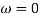). ). The exact resonance condition achieved when the frequency difference between the two light beams accurately matches with the frequency separation between the two lower states of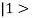and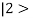.When the exact resonance condition is not achieved, the interference is not ideal the medium become absorbing.

Figure 5 represents the propagation of light in the EIT medium. At the beginning, the light pulse is outside the medium and all the atoms are in the ground states( |1>). The front edge of the light pulse then enters the medium and is quickly decelerated. The back edge of the light pulse propagates with vacuum speed c because it is still outside of the medium. Hence, upon entrance into the cell, the spatial extent of the light pulse is compressed by the ratio of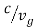, while its peak amplitude remains same. The energy of the light pulse is obviously much smaller when it is inside the medium. The photons are being expended to create the coherence between states of |1> and |2> , or in other words, to flip the atomic spins, with the excess energy carried away by the control field. Then, the wave of flipped spins now propagates together with the light pulse. The atoms are therefore strongly coupled to the photons which is in the light pulse, with an associated quasiparticle called a dark-state polarition. The quasiparticle is a combination of photons and spins excitation. The spatial extent increases again when the light pulse exits the medium and then the atoms return back to their original ground state. However, a delayed of the whole light pulse occurred by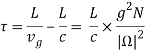(12)

where L is the length of the medium. In the dark-state polarition, when the control field is adiabatically switched off, the coupled excitation is converted into a pure atomic excitation, that is, the probe field is stopped. Then, to retrieve the probe field, the control field is switched on. A storage time of more than a second were achieved in this way.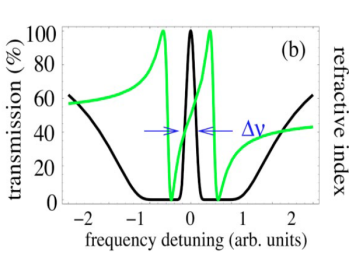Figure 4 : Spectrum of transmission and refractive index corresponding to EIT. This figure is taken from reference .

.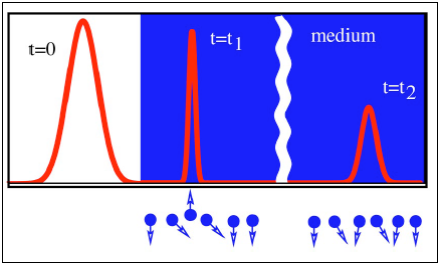Figure 4 : spatial compression occurred when the light beam enters the medium and at the same time the photons are changed into a atomic (spin excitation). This figure is taken from reference .

In the situation when the decay rate between stateandis negligible, the propagation of probe field can be describe by the electric field operator,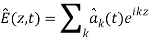(13)

where the sum is over the free-space of photonic modes with wave vectors k and bosonic operator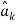. A collective atomic operators,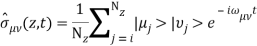(14)

averaged over small is used to describe the properties of the medium but at position z, macroscopic volumes containing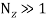particles .

Specifically, the operator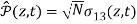is used to describes the polarization of atom oscillating at an optical frequency, whereas the operator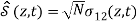decribes a low-frequency spin wave. Assumed that the control field is strong and it is treated classically. The atomic evolution is conducted by a set of Heisenberg equation which is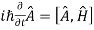, where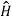is represent the atom-field interaction Hamiltonian and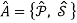. From these equations, the probe field is assuming to be weak and thatand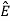change in time slowly. To leading order in the probe field, find that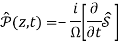(15)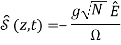(16)

The Heisenberg equation,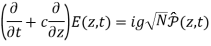(17)

described the evolution of the probe field. A new quantum field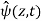which is a superposition of photonics and spin-wave components is introduced in order to attained the solution for the equations (15),(16) and (17).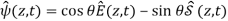(18)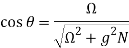(19)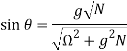(20)

Furthermore, the new quantum field,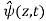must obeys the equation of motion,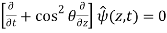. (21)

The equation (21) describes a shape-preserving propagation with velocity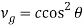which is proportional to the magnitude of its photonic component.

Moreover, EIT is also related to the Stark effect and Zeeman effects. Stark effects is the splitting of energy level due to the present of electric field while Zeeman effects is the splitting of energy level due to the present of magnetic field. If a volume is considered to be filled by a hydrogen atom and a DC field is applied to the volume, thestate will splits into three states with specific energies. By follow the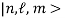notation ,the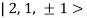states degenerates while the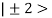states is generates which the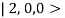state mixes with the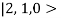states. The states are :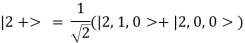(12)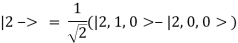(13)

Disscussion

In this section, I will disscuss about the implementation of EIT to observe the slow light and stopping.One of the technique used to induce transparency in opaque medium is “Coherent population trapping” or CPT. In CPT, the atoms are forced into a coherent superposition of Zeeman or hyperfine states that are strongly coupled to the light via Raman transition. The definition of superposition state is the state which is stable against absorption from the radiation of electromagnetic field[]. Generally, CPT is more refer to the concept of two fields closely equal to the Rabi frequency. The experiment done by Alexander et al.  used the concept of CPT to observe the slow light in a hot rubidium atoms. The main idea in the experiment is to obtain the narrow EIT resonance.

Our academic experts are ready and waiting to assist with any writing project you may have. From simple essay plans, through to full dissertations, you can guarantee we have a service perfectly matched to your needs.

Currently, the quantum mechanics is used for a fundamental of communication and computation. To carry out these ideas, information should be encoded in delicate quantum states, like a single-photon states, and then manipulated without being destroyed. It is fact that photons are the fastest and be a simplest carriers of quantum information. However, they are not easy to localize and process.

EIT has already had a large impact on the field of optical science. One of the potential application is used in optical buffering for the optical communication. From figure , there is an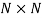switch in optical router. The switch can only deal with one data packet at a time . So if two data packet arrive at the same time, the collision of data packet will occur and the overall flow of the data packet will be slow down. Furthermore, the information carried by the two data packet will be loss. To avoid these problems, a slow light medium is activated in one of the branches in order to delay the velocity of data packet. As a result, the flow of information will be speed up because no collision between two data packet happened.

References

 G. Heinze, a. Rudolf, F. Beil, and T. Halfmann, “Storage of images in atomic coherences in a rare-earth-ion-doped solid,” Phys. Rev. A, vol. 81, no. 1, p. 011401, Jan. 2010.

 a. Turukhin, V. Sudarshanam, M. Shahriar, J. Musser, B. Ham, and P. Hemmer, “Observation of Ultraslow and Stored Light Pulses in a Solid,” Phys. Rev. Lett., vol. 88, no. 2, p. 023602, Dec. 2001.

 L. Hau, S. Harris, Z. Dutton, and C. Behroozi, “Light speed reduction to 17 metres per second in an ultracold atomic gas,” Nature, vol. 397, no. February, pp. 594–598, 1999.

 M. D. Lukin, “Colloquiumâ€¯: Trapping and manipulating photon states,” vol. 75, no. April, 2003.

 M. Lukin and A. ImamoÄŸlu, “Controlling photons using electromagnetically induced transparency,” Nature, pp. 273–276, 2001.

 M. Fleischhauer and M. Lukin, “Dark-State Polaritons in Electromagnetically Induced Transparency,” Phys. Rev. Lett., vol. 84, no. 22, pp. 5094–5097, May 2000.

 T. Chanelière, D. N. Matsukevich, S. D. Jenkins, S.-Y. Lan, T. a B. Kennedy, and a Kuzmich, “Storage and retrieval of single photons transmitted between remote quantum memories.,” Nature, vol. 438, no. 7069, pp. 833–6, Dec. 2005.

 a André, M. D. Eisaman, R. L. Walsworth, a S. Zibrov, and M. D. Lukin, “Quantum control of light using electromagnetically induced transparency,” J. Phys. B At. Mol. Opt. Phys., vol. 38, no. 9, pp. S589–S604, May 2005.

 S. Virally, “A Review of Slow Light Physics and Its Applications,” Ec. Polytech. Montr eal, pp. 82–90, 2008.

 D. Phillips, a. Fleischhauer, a. Mair, R. Walsworth, and M. Lukin, “Storage of Light in Atomic Vapor,” Phys. Rev. Lett., vol. 86, no. 5, pp. 783–786, Jan. 2001.

 M. Kash, V. Sautenkov, A. Zibrov, L. Hollberg, G. Welch, M. Lukin, Y. Rostovtsev, E. Fry, and M. Scully, “Ultraslow Group Velocity and Enhanced Nonlinear Optical Effects in a Coherently Driven Hot Atomic Gas,” Phys. Rev. Lett., vol. 82, no. 26, pp. 5229–5232, Jun. 1999.

 A. L. G. Robert W. Boyd, Daniel J. Gauthier, “Applications of Slow Light in Telecommunications,” Opt. Photonics News, vol. 17, no. April, p. 18, 2006.

View all

## DMCA / Removal Request

If you are the original writer of this essay and no longer wish to have your work published on UKEssays.com then please:

Related Services

Prices from

BHD57

Approximate costs for:

• 1000 words
• 7 day deliveryHumanity University

Dedicated to your worth and value as a human being!

Related Lectures